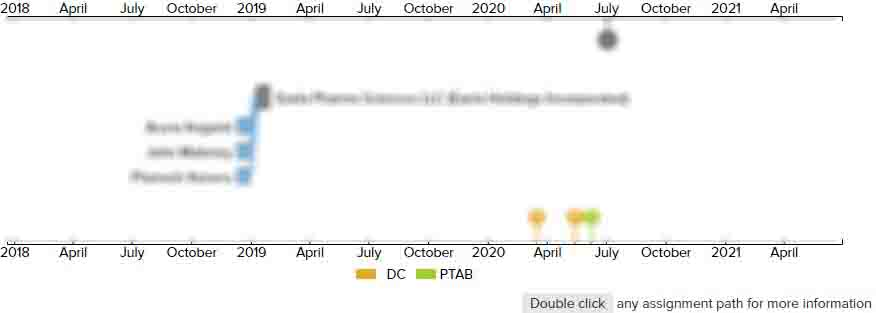# System and method for optimizing database queries

• US 5,822,747 A
• Filed: 08/23/1996
• Issued: 10/13/1998
• Est. Priority Date: 08/23/1996
• Status: Expired due to Term
##### First Claim
Patent Images

1. A method for generating a plan for executing a database query, comprising:

• (a) representing said database query as a query tree including one or more levels of expressions, each expression including zero or more expressions as inputs, each level of expression other than a top level expression having one or more expressions that are input to a higher level expression at a preceding level, the top level expression representing a root expression;

(b) storing in a memory a plurality of implementation and transformation rules, an implementation rule transforming a logical expression into a physical expression, a transformation rule transforming a logical expression into an equivalent logical expression;

(c) partitioning said query tree into one or more levels of subproblems, each subproblem including at least one logical expressions, a top subproblem level having one of said subproblems representing said root expression, each subproblem at each subsequent subproblem level representing one of said inputs to a corresponding higher level expression at a preceding level;

(d) determining an order for analyzing each of said subproblems, said order analyzing said subproblems from a lowest subproblem level to a top subproblem level such that each input is analyzed prior to its corresponding higher level expression;

(e) determining a first plan and a base cost for each subproblem, wherein the first plan comprises only physical expressions; and

(f) using said order, starting at said lowest subproblem level,(1) generating equivalent physical expressions for least one logical expression in each subproblem by applying at least one transformation rule and at least one implementation rule,(i) for each equivalent physical expression determine an associated equivalent-expression cost,(ii) if the equivalent-expression cost is less than the base cost, then denoting the associated equivalent physical expression as a plan and the equivalent-expression cost as the plan cost,(iii) selecting the plan with the lowest plan cost for use in a next higher subproblem level, and(2) repeatedly performing said generating step for higher subproblem levels until a plan is selected for said top subproblem level.

• ##### 6 Assignments
Timeline View
Assignment View×
×
×